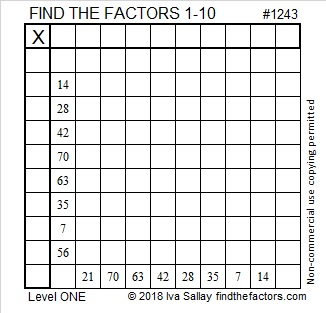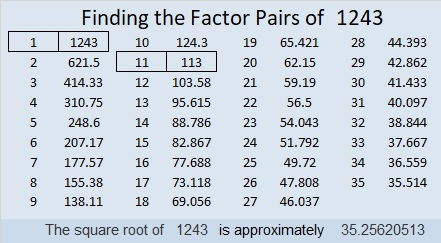# 1243 and Level 1

Here’s a great puzzle to help students figure out some division facts. That’s what they will have to do to find the factors from 1 to 10. Once they find those factors, they can complete the puzzle like it is a multiplication table.Print the puzzles or type the solution in this excel file: 10-factors-1242-1250

Now I’ll share a few facts about the number 1243:

• 1243 is a composite number.
• Prime factorization: 1243 = 11 × 113
• The exponents in the prime factorization are 1 and 1. Adding one to each and multiplying we get (1 + 1)(1 + 1) = 2 × 2 = 4. Therefore 1243 has exactly 4 factors.
• Factors of 1243: 1, 11, 113, 1243
• Factor pairs: 1243 = 1 × 1243 or 11 × 113
• 1243 has no square factors that allow its square root to be simplified. √1243 ≈ 35.256211243 is also the hypotenuse of a Pythagorean triple:
165-1232-1243 which is 11 times (15-112-113)

This site uses Akismet to reduce spam. Learn how your comment data is processed.